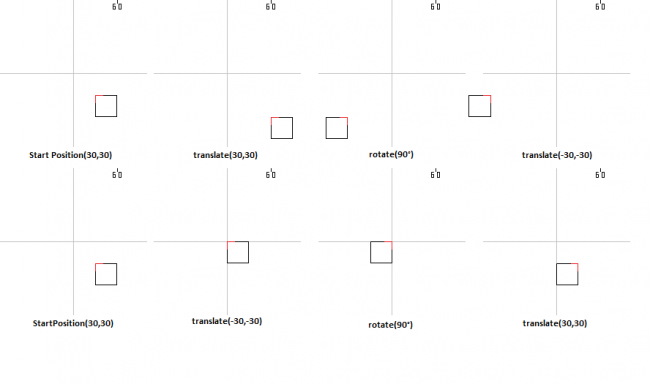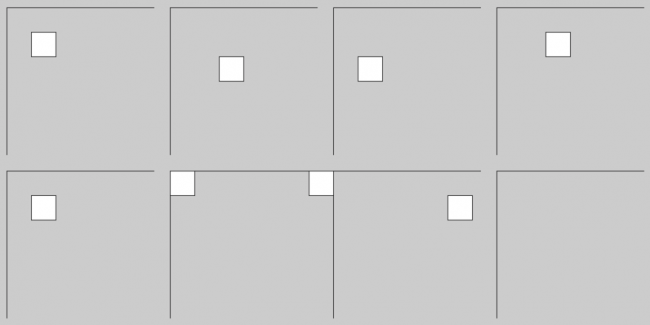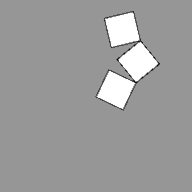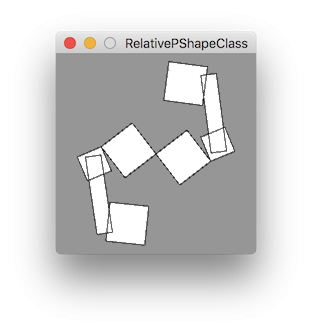#### Howdy, Stranger!

We are about to switch to a new forum software. Until then we have removed the registration on this forum.

# How to rotate a PShape relative to another?

edited May 2018

Hi, so at first this question might seem quite easy to answer (just use rotate()), but it's actually not what someone would expect. Rotate works based on the Origin(0,0), so if you are at (100,100) its still gonna rotate around that. So what is needed would be to translate(-x,-y), rotate(angle) and then translate back(x,y). This is all quite easy, but becomes impossible, without knowing the x or y coordinates in the first place... Now to my problem... What i have is a pretty complex hierarchycal Pshape(Groups)structure, so for simplicity, i made this, which basically explains the same situation, just more simple.

``````PShape GroupBoss;
PShape GroupVice;
PShape GroupMem;
PShape[] Low = new PShape;
PShape Target;
PShape Target2;
//Adjust Values here, to test as simple as possible^^ (took quite some time to get all done nicely xD)
float angle = radians(01); //just change this, so it rotates around it 0-360
int Child = 0; //Change to select next Child 0,1,2 (0 = no Child selected)
int Test = 0; //0 = test Child/s rotating relative to the parent; 1 = see Child not rotating relative to Parent rotating realtive to Parent (yeah, i did write it twice intentionally xD)
//No need to interfere with any Code other than above, to make Changes. Well... except for Code changes, but not for Testing around XD please use it a bit before finding the solution (as i said... took quite some time xD)

void setup() {
size(500, 500, P3D);
GroupBoss = createShape(GROUP);
GroupVice = createShape(GROUP);
GroupMem = createShape(GROUP);

for (int i = 0; i < Low.length; i++) {
Low[i] = createShape();
Low[i].fill(i*((205/3) + 50));
Low[i].vertex(0, 0);
Low[i].vertex(100, 0);
Low[i].vertex(100, 100);
Low[i].vertex(0, 100);
Low[i].endShape(CLOSE);
}

GroupBoss.translate(100, 200);
GroupBoss.getChild(0).translate(100, 0);
GroupBoss.getChild(0).getChild(0).translate(100, 0);
Target = GroupBoss;
for (int i = 0; i < Child; i++) {
Target = Target.getChild(0);
}
}

void draw() {
background(150);
shape(GroupBoss);
if (Test == 0) {
customRotate(Target, 0);
} else {
Target = GroupBoss.getChild(0);
Target2 = GroupBoss.getChild(0).getChild(0);
customRotate(Target, 0);
customRotate(Target2, 1);
}
}

void customRotate(PShape Shape, int test) {
Shape.translate(test == 0 ? -(Child * 100 + 100) : -(2*100+100), -200);
Shape.rotate(angle);
Shape.translate(test == 0 ? Child * 100 + 100 : 2*100+100, 200);
}
``````

It should be quite easy to understand where the problem lies, once you test the... test..., but just in case, i'll try to explain as detailed as i can. We have Pshape a<b<c with respective parents/childs (Parent<Child). Now if we rotate c, just c rotates like expected. If we rotate b, b and c rotate as expected together. But if we rotate b and c, b rotates like expected, but c (which should still rotate around the same axis as befor(in this case the top right corner of b))now rotates around the point where the top middle of b was before starting rotation... and i dont get why... so if anyone know why, or preferably a piece of code that solves this problem, please tell me... i'm looking for a workaround for at least 3 weeks now all over the internet and trying out everything i came up with... I'd also try complex math formuls, but since i cant access the x and y and angle of each shape, theres not much i can do... i also tried storing each x, y and ange translation/rotation in an extra PVertex/float array for each shape, but without much success(mainly cause it got a bit confusing xD)... Thanks^^

Edit: Btw, in some testing during the last weeks, i found out, that the relative position it (in this case c) rotates around, depends on the angle at which both rotate, respectively. So if both angles are equal,it goes where i said, but if one angle differs, the relative position around which c rotates changes... just like if it was changing it... but i dont know how i could counteract this. If i was able to do that, i could just come up with a way to offset it then, so that the relative position would be right again. Just said it, in case someone was gonna say, to just add x + 25 to the offset (in this case). Because even though it works here with the fixed position, it wouldnt if the angles would differ.

Tagged:

• edited May 2018 Answer ✓

Uhm... i don't get it at all... but i just found a solution? i think?... basically this works... :

``````PShape[] Shapes = new PShape;
PShape[] Groups = new PShape;

void setup() {
size(600,600);
Shapes = createShape(RECT, 30,30,30,30);
Shapes = createShape(RECT, 60,60,30,30);
Shapes = createShape(RECT, 90,90,30,30);
Groups = createShape(GROUP);
Groups = createShape(GROUP);
Groups = createShape(GROUP);
}

void draw() {
background(150);
Groups.translate(60,60);
Groups.translate(-60,-60);
shape(Groups);
Groups.translate(90,90);
Groups.translate(-90,-90);
}
``````

but i dont know why... it should be the exact opposite... right? anyway, to have to set the start positon manually is kinda wierd. If someone from Processing sees this, please add it, so that this origin this is obsolete. Just add a second method that is like

``````rotate(float angle,boolean origin);
if (origin) {
translate(-m00, -m01); //cause i know there are some position variables, just that they are protexted, while the accessable X,Y,Z variables always return 0...
rotate(angle);
translate(m00,m01);
}
``````

or something like that. I'm sure for advanced programmer thats nothing xD but it causes some problems, if you dont know about it and are not overly familiar with it^^. Well, anyways... with this the question is solved... even if i still dont know why xD. So, if someone knows why, please tell me, even if it's set as answered^^

• edited May 2018 Answer ✓

It looks like the key thing for you to understand here is that the PShape matrix (translate, rotate, etc.) is cumulative -- unlike in the main sketch, which is reset at the end of each draw.

So you are doing this right -- place each joint relative to where you want them connected, then move to that joint (translate out), spin the object on its axis (rotate), and place the spun object relative to the parent image (translate back).

One minor confusion is that you seem to think that what you are doing in setup is placing things absolutely on the canvas -- for example, you start the first shape at 30,30. This isn't really what you are doing -- you are placing them relative to one another. It is a bit clearer if you factor that 30,30 out of everything, then make your placement of the object a separate operation -- that absolute layout data shouldn't be in the PShape at all, only relative distances.

``````PShape[] Shapes = new PShape;
PShape[] Groups = new PShape;

void setup() {
size(300, 300);
Shapes = createShape(RECT, 0, 0, 30, 30);
Shapes = createShape(RECT, 30, 30, 30, 30);
Shapes = createShape(RECT, 60, 60, 30, 30);
Groups = createShape(GROUP);
Groups = createShape(GROUP);
Groups = createShape(GROUP);
}

void draw() {
background(150);
translate(mouseX, mouseY);
Groups.translate(30, 30);
Groups.translate(-30, -30);
Groups.translate(60, 60);
Groups.translate(-60, -60);
shape(Groups);
}
``````

Okay, now try separating the idea of an axis of rotation from the idea of your shape. It just so happens that your shapes always have their axis of rotation on one corner, but that is a numeric coincidence -- they aren't logically related. Define your axes in a separate list -- one per group.

``````PShape[] Shapes = new PShape;
PShape[] Groups = new PShape;
PVector[] Axes = new PVector;

void setup() {
size(300, 300);
Shapes = createShape(RECT, 0, 0, 30, 30);
Shapes = createShape(RECT, 30, 30, 30, 30);
Shapes = createShape(RECT, 60, 60, 30, 30);
Axes = new PVector(0, 0);
Axes = new PVector(30, 30);
Axes = new PVector(60, 60);
Groups = createShape(GROUP);
Groups = createShape(GROUP);
Groups = createShape(GROUP);
}

void draw() {
background(150);
translate(mouseX, mouseY);
for (int i=0; i< Groups.length; i++) {
Groups[i].translate(Axes[i].x, Axes[i].y);
Groups[i].translate(-1 * Axes[i].x, -1 * Axes[i].y);
}
shape(Groups);
}
``````

Now notice that you can change your shape definitions into whatever you want -- for example, making them different sizes or giving them borders. The underlying armature of linked axes remains the same -- it doesn't have anything to do with the drawing.

``````  Shapes = createShape(RECT,-15,-15, 60, 60);
Shapes = createShape(RECT, 25, 25, 40, 40);
Shapes = createShape(RECT, 55, 55, 40, 40);
``````

At this point you have parallel arrays -- your groups array, and your axes array. If you wanted you could evolve this into a class. Right now your series of axes is a linear chain -- Group 1, 2, 3 -- but you could also make it branching, as with a body that has four limbs, and each limb has a hand or foot.

• Sorry for the loooong Text^^ ended up being more than i thought. (might sound bad sometimes, but thats not intentional (just dont know how to say stuff xD)

• @Lexyth -- not harsh, and I'm not offended.

You stated twice that your solution functioned, but you did not understand why it works.

Uhm... i don't get it at all... but i just found a solution? i think? [...] the question is solved... even if i still dont know why

You requested follow-up explanation. So I broke down the functionality in your solution and tried to explain why it works as it does. Sorry if I guessed wrong that you were unclear on the cumulative part -- it is hard to know what you mean by "i don't get it at all".

You objected that :

i already got the same result when i posted the other answer [...] the functionality of both sketches is pretty much identical

Yes, that's because it is your code with minor changes to illustrate how your solution already works -- in particular, how the axis or rotation work independent of the drawing coordinates. It doesn't have any different functionality. You didn't ask for a different solution, you asked why your own solution works.

No reason you can't continue this thread with follow up questions about 3D. That is welcome here, and keeping it in one thread is better than making a new one about almost the same code -- this isn't like Stackoverflow.

• Thanks^^ actually with "i dont get it at all" i was referring at the fact, that first comes the positive translation, then the negative translation (that minus comes first, not that it has to get translated and then put back to the location it was, even though i still think that should be included in rotate(), or at least in another method called rotateAtPosition() or so... Or actually to also add another method callse rotateAroundPoint()... but i guess there are reasons...? ) because what i would assume after doing this, would be the following Positions ==> Start at 30,30 translate(30,30); Position would be now 60,60... rotate(radians(90)); Since rotation goes around the Origin it would put the corner at -60,60... if im not totally wrong (might be a bit differen number, but if i got right how a matrix rotation works, that should be right... (or actually just thinking about it, it should be right... )) translate(-30,-30); Position would now be -90,30 note, that the position im talking about is actually just the top left corner. while doing the opposite : Start 30,30 translate(-30,-30); Position 0,0 rotate(radians(90)); Position is 0,0 of the left corner, which know would have become the top right corner translate(30,30); position is now back to 30,30 with a rect that is rotated by 90°.

I'm actually gonna make a picture in paint, just to see if i really didnt get something wrong and post that too... :Hope this isn't too small... (Edit: it's fine i guess^^)

Note, that the picture if left to rigth and the upper is how the numbers go in processing, while the lower is how i would think its should work (and as seen, it works contrary to the one that works in processing, but not in theory xD) But i might (probably) just have gotten something extremely wrong or so, but i just cant figure out what it is.

Oh, and also about the 3D version, yeah, it would be really great is you'd know a way to only get this sketch in a 3D version. So that i can at least get the way to get it to work (preferably understand it, but i can also just apply it, if i dont get it, sooo... XD) . Because currently i downloaded a sketch from github, in which the 3D movement works, and am trying to dismantle it, so i get to understand how it could work, because what i currently get from that code is not too much... quite confusing, and i gotta look at everything, since i dont know where the code actually is and that is quite a lot of entangled code xD (7 files with average 300 lines...) Anyway XD Thanks again for the fast answer^^ (and sorry for the late answer... thought paint would take 10 minutes... one hour ago...)

• Both approaches work fine, except your eighth image is a mistake.

It looks like in image 8 you are expecting translate(30,30) to apply an absolute translation in terms of the original reference frame, but the reference frame is now rotated 90 degrees, so 30,30 now points southwest, not southeast.

So, you said:

position is now back to 30,30

...but if you are at 0,0 and rotated 90 then translating 30,30 takes you to -30,30.

Luckily this is easy to experiment with in Processing.

``````PShape aShape;

void setup() {
size(800, 400);
aShape = createShape(RECT, 0, 0, 30, 30);
aShape.translate(30,30);
}

void draw() {
translate(10,10);
graph(0,0);
aShape.translate(30,30);
graph(200,0);
aShape.rotate(HALF_PI);
graph(400,0);
aShape.translate(-30,-30);
graph(600,0);

aShape.resetMatrix();

aShape.translate(30,30);
graph(0,200);
aShape.translate(-30,-30);
graph(200,200);
aShape.rotate(HALF_PI);
graph(400,200);
aShape.translate(30,30);
graph(600,200);
noLoop();
}

void graph(float x, float y) {
pushMatrix();
translate(x, y);
line(0, 0, 0, 180);
line(0, 0, 180, 0);
shape(aShape);
popMatrix();
}

void drawAxes() {
}
``````I know you said you understand that the matrix is cumulative, but it may be helpful for you to review the examples in the 2D Transformations tutorial...

• Okay... i looked at the tutorial, and i think i got it now. I knew that the translation moves the grid, but apparently i just didnt think about it xD after looking at it again and keeping that in mind, i actually found the mistake too xD Though i still have a problem i cant see the solution to in the tutorial... and that is to just do exactly the same in P3D... once P3D is active, it just workes completely different... even if you just use exactyl the same code...

• it just works completely different

What do you mean, exactly? And what do you mean by "exactly the same"? Can you give a simple specific example? The camera and perspective means that thing won't line up in exactly the same way as 2D, but the rules should be the same.

• Just use this code and remove and add again P2D and it will completely change, without changing the code. (without p2d it works... but its still both even 2D... so i dont get why it changes the way it works xD) and the problem is that i need p3d to shape 3D shapes, but i cant even figure out how to rotate in p2d, less p3d)

``````PShape[] Shapes = new PShape;
PShape[] Groups = new PShape;
PVector[] Axes = new PVector;

void setup() {
size(300, 300, P2D);
Shapes = createShape(RECT, 0, 0, 30, 30);
Shapes = createShape(RECT, 30, 30, 30, 30);
Shapes = createShape(RECT, 60, 60, 30, 30);
Axes = new PVector(0, 0);
Axes = new PVector(30, 30);
Axes = new PVector(60, 60);
Groups = createShape(GROUP);
Groups = createShape(GROUP);
Groups = createShape(GROUP);
}

void draw() {
background(150);
translate(mouseX, mouseY);
for (int i=0; i< Groups.length; i++) {
Groups[i].translate(Axes[i].x, Axes[i].y);
Groups[i].translate(-1 * Axes[i].x, -1 * Axes[i].y);
}
shape(Groups);
}
``````
• I don't know... i got another "solution" to get it to work with P2D&P3D, but it's not really simple... you basically gotta add an extra class for it to work... it looks bigger than it is, but i'll comment on what is not needed for the class(i'll just leave it, if someone wants it)... there might be an easier way, but i just cant see it... //not needed // means that its not needed for it to just work.

``````class Component {
float angle; // the angle it has currently / can be changed to a vector, but will need further changes in the code
float maxAngle, minAngle; //not needed
float targetAngle; // the angle it has to go to
float angularVelocity; //not needed if you want it to jump to the targetangle/can be set to 1;
float maxVelocity; //not needed
String name; //not needed

PShape shape; //the shape it has to display
float xPos; //the current position (relative to the parent(will be explained in the
draw method))
float yPos; //same as xPos, just on y
float zPos = 0; // same as xPos, just on z (not used in the code, but can easily be added
//can also be made into a PVector

Component(PShape shape_, float xPos_, float yPos_, float angle_, float minAngle_, float maxAngle_, float maxVelocity_, String name_) { //should be obvious
angle = angle_;
targetAngle = angle_;
minAngle = minAngle_;
maxAngle = maxAngle_;
angularVelocity = 0;
maxVelocity = maxVelocity_;
name = name_;
shape = shape_;
xPos = xPos_;
yPos = yPos_;
//obvious too
}

boolean active() { // get if the PShape is already being rotated at the moment
if (angularVelocity != 0) {
return true;
} else {
return false;
}
}

void setAngle(float newAngle) { //set an angle
angle = newAngle < minAngle ? minAngle : newAngle > maxAngle ? maxAngle : newAngle;
angularVelocity = 0;
}

void setPosition(float xPos_, float yPos_) { //set a specific position (may be relative)
xPos = xPos_;
yPos = yPos_;
}

void write(float newAngle, float newVelocity) { // set the targetangle
if (newAngle != angle) { // angle is not already same
targetAngle = newAngle < minAngle ? minAngle : newAngle > maxAngle ? maxAngle : newAngle; //not needed if no minMax is included /makes sure angle is not smaller than min or max
newVelocity = abs(newVelocity); //make sure velocity is positive
newVelocity = newVelocity > maxVelocity ? maxVelocity : newVelocity; //check if is smaller than maxVel
angularVelocity = targetAngle < angle ? -newVelocity : newVelocity; //check if smaller than angle
}
}

void update() { // update the angle
if (active()) {
if (abs(targetAngle-angle) < abs(angularVelocity)) {
angle = targetAngle;
angularVelocity = 0;
} else {
angle += angularVelocity;
}
}
}

void display() { //display the shape at position
translate(xPos,yPos,0);
rotateX(0);//can be changed to angle.x, if PVector is used
rotateY(0);
rotateZ(angle);
shape(shape); //draw the shape
}
}
``````

//now on to the main functions

``````int n; //to increase angle / not needed if option 2 is used (will come in the draw function
int Size = 5; //sets the amount of components used
PShape[] shapes = new PShape[Size];
Component[] comps = new Component[Size];
PVector[] pos = new PVector[Size]; //where to display() the shapes

void setup() {
size(500,500,P3D);
pos = new PVector(0,0);
pos = new PVector(-30,0);
pos = new PVector(30,0);
pos = new PVector(-30,30);
pos = new PVector(30,30); //manual positioning of the components

for (int i = 0; i < Size; i++) {
shapes[i] = createShape(RECT,0,0,30,30); // create the shapes
comps[i] = new Component(shapes[i],30,30,0,0,radians(360),1,str(i)); //create the components
}
}

void draw() {
graphics(); //draw the graphics
n++; //increase rotation angle //option2: dont use it
for (int i = 0; i < Size; i++) {
}
comps.setPosition(mouseX,mouseY); //set position of main comp at mouse
pushMatrix(); //used to specify if comps are relative to eachother (example will come later)
for (int i = 0; i < Size; i++) { //draw each shape at postition
comps[i].display();
}
popMatrix(); //same use as pushmatrix
//Add user controls //still need to do that... basicaly just at mouse pressed, write for selected component exm: if (mousepresses at 200,200) comps[i].write(PI, radians(1));
}

void graphics() { //graphics
background(50);
translate(width/2, height/2, 0); //set shape in middle of screen
}
``````

example for pushMatrix :

``````pushMatrix();
comps.display(); //will be drawn at starting pos (in this case mouseX, mouseY)
comps.display(); //will be drawn relative to 
comps.display(); //will be drawn relative to 
popMatrix();
pushMatrix();
comps.display(); //will be drawn at starting pos
popMatrix();
pushMatrix();
comps.display(); //will be drawn at starting pos
popMatrix();
``````

this should be all... hope its clear and that i didn't forget anything^^ also, while posting, i noticed, that it could actually be way shorter, but since i wanted to make it fast to use, i let it be like this, to save others time including the methods i already had in it xD. Anyway^^ hope it helps, if someone else had problems with this too^^

• An alternate approach if you want something simple that works the same way in JAVA2D, FX2D, P2D, or P3D. Does not require groups.

1. Store basic shapes centered on 0,0.
2. Store a chain of shape relationships (x, y, rotation) in a PVector array as relative offsets.
3. Look through shapes, updating each offset and performing a transform on the basic drawing matrix.

Like this:

``````import peasy.PeasyCam;
PeasyCam cam;

PShape[] shapes;
PVector[] offset;
void setup() {
size(200, 200, P2D); // works with JAVA2D, FX2D, P2D, or P3D
cam = new PeasyCam(this, 300);
shapes = new PShape;
offset = new PVector;
shapes = createShape(RECT, 0, 0, 30, 30);
shapes = createShape(RECT, 0, 0, 30, 30);
shapes = createShape(RECT, 0, 0, 30, 30);
offset = new PVector(0, 0, 0);
offset = new PVector(30, 30, 0);
offset = new PVector(30, 30, 0);
}
void draw() {
background(150);
translate(width/2, width/2);
pushMatrix();
for (int i=0; i< shapes.length; i++) {
translate(offset[i].x, offset[i].y);
rotate(offset[i].z);
shape(shapes[i]);
}
popMatrix();
}
``````• Belatedly: because you also shared a class-based solution, here is simplified class-based solution which uses the global PMatrix -- it also works equally well in JAVA2D, FX2D, P2D, or P3D (although peasycam only works in P3D).

One limitation of this approach: it can only go to the depth of the global PMatrix. If you nest a large set of shapes in a long chain then you could run out of stack and the sketch will stop with an error. However for paper dolls, robot arms, or spiders et cetera this approach works great.

``````// RelativePShapeClass
// Jeremy Douglass 2018-05-14 Processing 3.3.6
// forum.processing.org/two/discussion/27880/how-to-rotate-a-pshape-relative-to-another

import peasy.PeasyCam;
PeasyCam cam;

void setup() {
size(200, 200, P3D); // works with JAVA2D, FX2D, P2D, or P3D
cam = new PeasyCam(this, 300);
createShape(RECT, 0, 0, 30, 30),
new PVector(0, 0, 0),
new PVector(0, 0, 0.5));
createShape(RECT, 0, 0, 30, 30),
new PVector(0, 0, 180),
new PVector(0, 0, 0.5));

createShape(RECT, 0, 0, 20, 20),
new PVector(30, 30, 0),
new PVector(0, 0, 0.5));
createShape(RECT, -6, -6, 12, 60),
new PVector(10, 10, 0),
new PVector(0, 0, 0.5));
createShape(RECT, 0, 0, 30, 30),
new PVector(0, 60, 0),
new PVector(0, 0, 0.5));
shapes = ls0;
shapes = ls1;
}

void draw() {
background(150);
// translate(width/2, width/2);
pushMatrix();
for (int i=0; i< shapes.length; i++) {
shapes[i].display();
}
popMatrix();
}

PShape ashape;
PVector offset;
PVector movement;
LinkedShape(PShape ashape, PVector offset, PVector movement) {
this.ashape = ashape;
this.offset = offset;
this.movement = movement;
}
void update() {
}
void update(PVector m) {
}
void display() {
pushMatrix();
this.update();
translate(offset.x, offset.y);